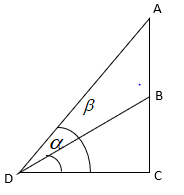Questions & AnswersQuestionAnswers

# A vertical tower stands on a horizontal plane and is surmounted by a flag-staff of height 7m from a point on the plane, the angle of elevation of the bottom and the top of flag-staff are ${{30}^{0}},{{45}^{0}}$ respectively. Find the height of the tower.Answer Verified
Hint:To solve the question, we have to apply trigonometry rules for the figure drawn from interpreting the given information.

Complete step-by-step Solution:
Given
The height of the flag-staff surmounted on vertical tower = 7 metres
Let AB be the height of the flag-staff and BC be the height of the vertical tower and $\alpha ,\beta$be the angle of elevation of the bottom and the top of flag-staff respectively.AB = 7 metres and AC = AB + BC = (7 + BC) metres.
The given angle of elevation of the bottom of the flag-staff = ${{30}^{0}}$
The angle between BD and AC is equal to ${{30}^{0}}$
$\Rightarrow \alpha ={{30}^{0}}$
The given angle of elevation of the top of the flag-staff = ${{45}^{0}}$
$\Rightarrow \beta ={{45}^{0}}$
The angle between AD and AC is equal to ${{45}^{0}}$
By applying trigonometry of angles, we get
$\tan \alpha =\dfrac{BC}{CD}$
$\tan \beta =\dfrac{AC}{CD}$
By substituting the angles and values in the above formula we get,
$\tan {{30}^{0}}=\dfrac{BC}{CD}$
$\tan {{45}^{0}}=\dfrac{7+BC}{CD}$
We know that the values of $\tan {{45}^{0}}$is equal to 1 and the value of $\tan {{30}^{0}}$is equal to $\dfrac{1}{\sqrt{3}}$.
$\Rightarrow \dfrac{1}{\sqrt{3}}=\dfrac{AC-7}{CD}$ and $1=\dfrac{AC}{CD}$
$\Rightarrow CD=\sqrt{3}BC$ and $CD=7+BC$
By solving the above expression, we get
$\sqrt{3}BC=7+BC$
$BC\left( \sqrt{3}-1 \right)=7$
$BC=\dfrac{7}{\left( \sqrt{3}-1 \right)}=\dfrac{7}{1.73-1}=\dfrac{7}{0.73}=9.58$metres
Thus, the height of the tower is equal to 9.58 metres.
Note: The possibility of mistake can be not applying trigonometry for solving the problem. The alternative way for easing the procedure is by using other cosine, sine, cot angles for solving. The other alternative quick way of solving is applying the direct formula for the height of the tower $\dfrac{x\tan \alpha }{\left( \tan \beta -\tan \alpha \right)}$ where x, are height of the flag-staff, the angle of elevation of the bottom and the top of flag-staff respectively.
Bookmark added to your notes.
View Notes
Visualising Circular Motion in Vertical PlaneReflection on A Plane MirrorAngle Between a Line and a PlaneAdjacent and Vertical AnglesMotion in a PlaneWhat is a Plane?Equation of a PlanePerpendicular Distance Of A Point From A PlaneGeneral Equation of a PlaneVertical Line